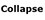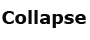# PH 205

## Kepler's laws

Understanding some basic physics, including Kepler's laws, will be important in understanding topics like galaxy rotation and the discovery of dark matter. In the 17th century, Johannes Kepler made a major contribution to understanding the dynamics of astronomical bodies by analyzing a lifetime of observations take by his mentor, Tycho Brahe. These observations included decades of observing the positions of the planets against the background of stars. He recognized that there was a pattern associated with the orbital periods of the planets, and used this knowledge to determine the relative sizes of the planets orbits about the sun. He found the orbital speeds of the planets by comparing their motions over time.

Kepler's discoveries can be summarized in three laws.

Kepler's first law

Planet orbits are ellipses with the sun at one focus of the ellipse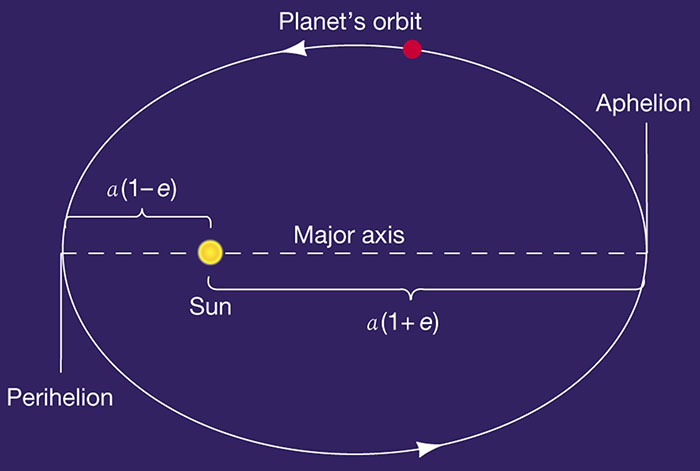A circle is a special case of an ellipse, with only one focal point. An ellipse typically has two focal points. The farther apart the focal points, the greater the ellipticity of the ellipse. The "diameter" across the long side is called the major axis. The semi-major axis (a) is one-half the major axis. This diagram is greatly exaggerated, most planet orbits in our solar system are nearly circular.

## Kepler's second law

A line connecting a planet to the Sun sweeps out equal areas in the ellipse in equal times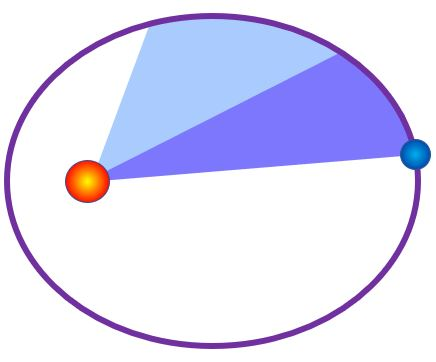A planet moves faster when it is closer to the sun and slower when it is farther away. In the image above, the same amount of time elapsed while tracing out the blue area as the purple area.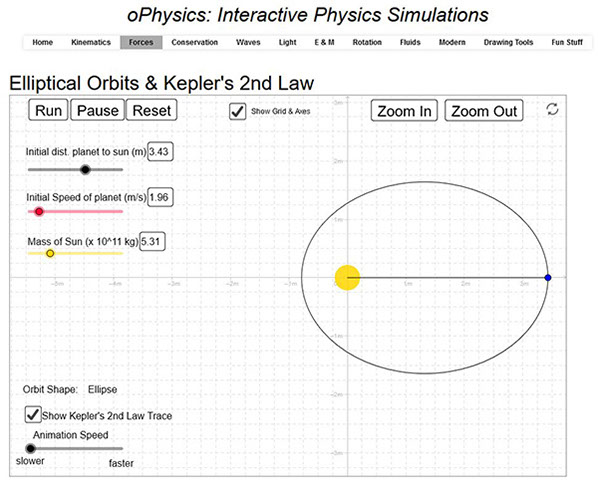Please check out this oPhysics interactive illustrating Kepler's second law. Adjust the initial speed of the planet to change the ellipticity of its orbit.

## Kepler's third law

The square of the orbital period of a planet equals the cube of its semi-major axis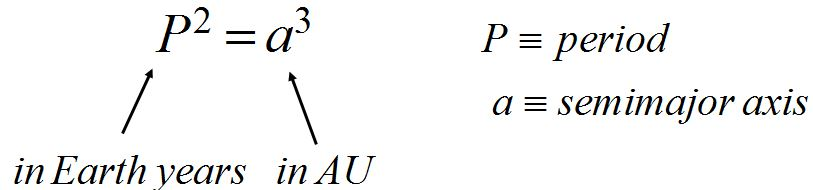Since the orbits of the planets are nearly circular, you can think of the semi-major axis of the ellipse as the radius of the planet's orbit. Since the sun's mass is so much larger than the planets' masses, you often see this equation written without the Mtotal term.

The Astronomy Interactives simulation titled "Kepler's Third Law Interactive" gives a good visualization of this law of planetary motion.

With these three laws, the motions of the planets were understood in terms of the orbital distance of the earth, or in units of AU's. The true distances could not be found until a method was devised to make a measurement between two objects in our solar system to set the scale. Using parallax methods, astronomers were able to calculate the distance between Earth and Venus, and use geometry to figure out the difference of the orbital radii of these two planets. We were later able to check this calculation using radar-ranging. Impressively, we were able to bounce a radar signal off the thick atmosphere of Venus. Knowing the speed of light allowed us to directly measure the distance between the two planets.

## Newton's laws

Isaac Newton revolutionized the understanding of the behaviors of massive objects. Before his day, people believed that the natural state of an object was to be at rest, as close to the center of the universe (the earth) as possible. They thought that if you hit an object, you gave it energy. The energy would be used up, and the object would go back to its natural state of rest. It is understandable that people thought this way, because that is what most objects seem to do. Newton developed a new way of understanding the mechanics of motion, which can be summed up in three laws.

Newton's first law

An object at rest stays at rest. An object in motion stays in motion at a constant speed in a straight line unless acted upon by an unbalanced force.This introduced the notion of a force. The mathematical relationship deriving the nature of forces was defined in Newton's second law. Even so, the first law states something fundamental about the behavior of objects. An object doesn't have a natural state of rest.

Newton's second law

The net force on an object is equivalent to the product of object's mass and its accelerationIn the image above, the donkey has three forces acting on it. There is a push from behind, a pull from the rope, and a push backward from the ground. Since the donkey is not changing its velocity, the net force must be zero.

There is one more thing to consider here. We need to define what mass means. Mass is related to weight. Weight is defined as mass x acceleration due to gravity. Acceleration due to gravity is often represented by the letter g. mathematically we would write

W = mg

Now the acceleration due to gravity is pretty nearly constant on the surface of Earth, but it is very much different, say, on the moon. Your mass is the same on Earth and on the moon, but your weight is very different.

Newton's third law

Forces come in pairs. For every force, there exists and equal and opposite force.

In the case of the force on the donkey's feet from the ground, there is an equal and opposite force on the ground from the donkey's feet. For a hammer hitting a nail, the force felt by the nail from the hammer is equal and opposite to the force felt by the hammer from the nail. Newton's third law force pairs are always felt by the two objects in question. Using the notation Fon,from, we can write this law as F12 = -F12.

What are the forces in the Newton's 3rd law force pair below?If you said that the force felt by the man from the rock was equal and opposite to the force felt by the rock from the man, you were right. Fman,rock = -Frock,man. The minus sign indicates that the two forces are in opposite directions.

What about the case shown below? The ball feels a gravitational force from Earth. What is the Newton's third law pair force?The opposing Newton's third law force is the gravitational force felt on the earth from the ball. The ball and Earth are both massive objects, and they exert gravitational forces on each other. The ball undergoes an acceleration of g, or 9.8 m/s2. The earth undergoes an acceleration too, but it is much smaller since the mass of Earth is so much greater.

Fball,earth = -Fearth,ball

ma = -ma

There is a common saying, "for every action there is an equal and opposite reaction." It sounds like a restatement of Newton's third law, but it is often meant to mean something quite different. Newton's third law is very specific to forces and doesn't hold for other quantities in general.

•